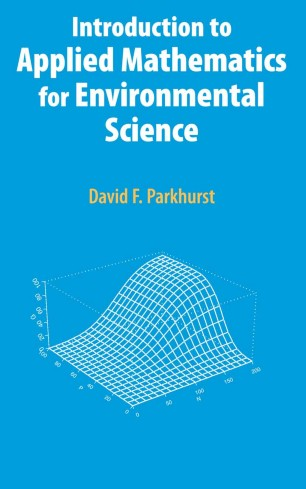# Introduction to Applied Mathematics for Environmental Science

• David F. ParkhurstTextbook

1. Front Matter
Pages i-ix
2. Pages 1-18
3. Pages 19-51
4. Pages 52-81
5. Pages 82-122
6. Pages 123-136
7. Pages 137-146
8. Pages 166-192
9. Pages 193-228
10. Pages 229-249
11. Pages 250-284
12. Back Matter
Pages 285-317

### Introduction

Introduction to Mathematics for Environmental Science evolved from the author’s 30 years’ experience teaching mathematics to graduate and advanced undergraduate students in the environmental sciences. Its basic purpose is to teach various types of mathematical structures and how they can be applied in a broad range of environmental science subfields. Derivatives and integrals, ordinary and partial differential equations, and linear and non-linear algebraic equations are the basic kinds of structures (types of mathematical models) discussed.

For each mathematical topic, the book emphasizes derivation of mathematical models from "story problems" first, and solution of equations second. It suggests finding analytic solutions when possible, but discusses methods for finding numerical solutions to problems that can’t be solved analytically. For most topics, examples of using Matlab software to solve and explore the structures are also included.

This text assumes that its readers will already have been introduced to the basic ideas of differential and integral calculus, so it is not an introduction to calculus. It does, however, include three early chapters to review basic algebra, derivatives, and integrals.

Professor Parkhurst earned his B.S. degree in applied mathematics from the College of Engineering, University of Colorado, Boulder, in 1965, and his Ph.D. in Botany (Plant Ecology) from the University of Wisconsin, Madison, in 1970. He then worked three years at the CSIRO Division of Atmospheric Science in Aspendale, Victoria, Australia, before joining the Environmental Science faculty of the School of Public and Environmental Affairs, Indiana University, Bloomington, in 1973. He held a joint appointment in the Biology Department there as well. He retired from that position in December, 2005, after 32 years. His research areas include mathematical modelling of adaptations of plant leaves to environment, environmental risk analysis, and applications of statistics in environmental and public health areas.

### Keywords

MATLAB algebra environmental science linear algebra mathematics ordinary differential equation partial differential equation

#### Authors and affiliations

• David F. Parkhurst
• 1
1. 1.Indiana UniversityBloomington